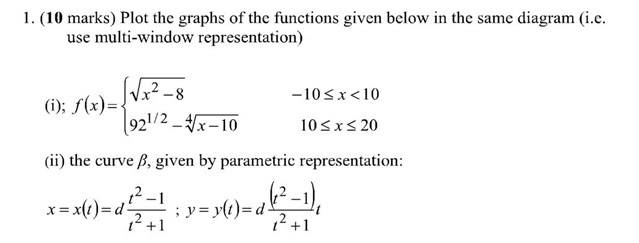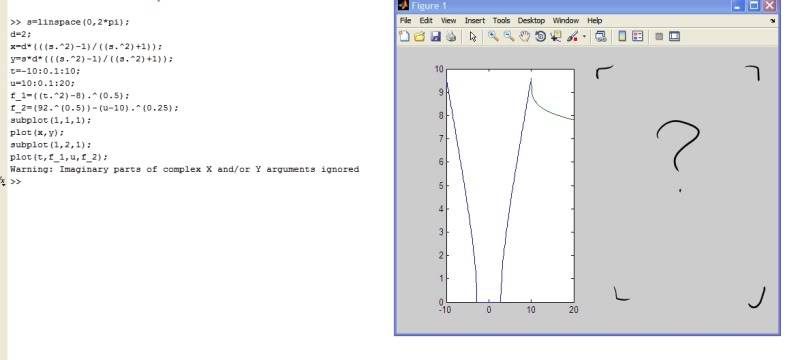# Bit of help with piecewise and parametric functions in MATLAB?

• MATLAB
• korobeiniki
In summary, the conversation discusses a user's experience with using a forum for help, deciding to make an account to potentially contribute, and being assigned an assignment for MATLAB. They mention being stuck on question one and not being satisfied with their code, specifically a piecewise function. The user then edits their post to say they have figured out the issue and thanks the forum. They also mention the helpfulness of the MATLAB web documentation.

#### korobeiniki

Hi guys, after using the forum for ages for help I decided to make an account, so hopefully I might be able to contribute something as well, who knows :)
I'm a first timer with MATLAB and have been set an assignment. I'm completely stuck on question one:The following image shows my code and the resulting figure. For some reason the second (parametric) graph is missing:Also I'm not really happy with the code - the first function is piecewise but I've written it out as 2 separate functions. Is there some way to define this as one, piecewise, function?

Edit!: cracked it! my subplot command was all wrong: should've had (1,2,1) and (1,2,2) respectively. Also I was using matrix operators rather than array operators for the parametric curve. Thanks anyway!

Last edited:
Welcome to PhysicsForums!

First off, place code in-between [CODE ][\CODE ] brackets (remove the space before the final bracket: it maintains your white space and helps increase readability.

Secondly, especially as you're not doing this using function handles, it suffices to treat piece-wise functions, well, piece-wiseNote that you've also got the datapoint at 10 twice (which is okay, since you're not concatenating the two vectors). To concatenate them, just do something like the following, which will put the two vectors together (x1 values followed by x2):

>>x=[x1, x2];

Good for you for figuring out the rest of it! For future reference, the MATLAB web documentation is really, really complete and helpful (even more so than the generic help (function) command in MATLAB):
http://www.mathworks.com/help/techdoc/creating_plots/f6-20079.html#f6-35125 [Broken]

Last edited by a moderator:

## 1. What is a piecewise function in MATLAB?

A piecewise function in MATLAB is a function that is defined by multiple sub-functions, with each sub-function being applicable to a certain interval of the independent variable. This allows for different expressions to be used for different parts of the input range.

## 2. How do I define a piecewise function in MATLAB?

To define a piecewise function in MATLAB, you can use the "piecewise" function or the "if" statement. The "piecewise" function allows you to specify the different sub-functions and their corresponding intervals, while the "if" statement allows you to specify conditions for each sub-function.

## 3. What is a parametric function in MATLAB?

A parametric function in MATLAB is a function that is defined by one or more parameters. These parameters can take on different values, which can change the behavior of the function.

## 4. How do I define a parametric function in MATLAB?

To define a parametric function in MATLAB, you can use the "syms" function to define symbolic variables, and then use these variables in your function definition. This allows you to easily change the parameters and see how it affects the function.

## 5. Can I use piecewise and parametric functions together in MATLAB?

Yes, you can use piecewise and parametric functions together in MATLAB. You can define a piecewise function with parameters, or you can use a parametric function within a piecewise function. This allows for more flexibility in your function definitions.